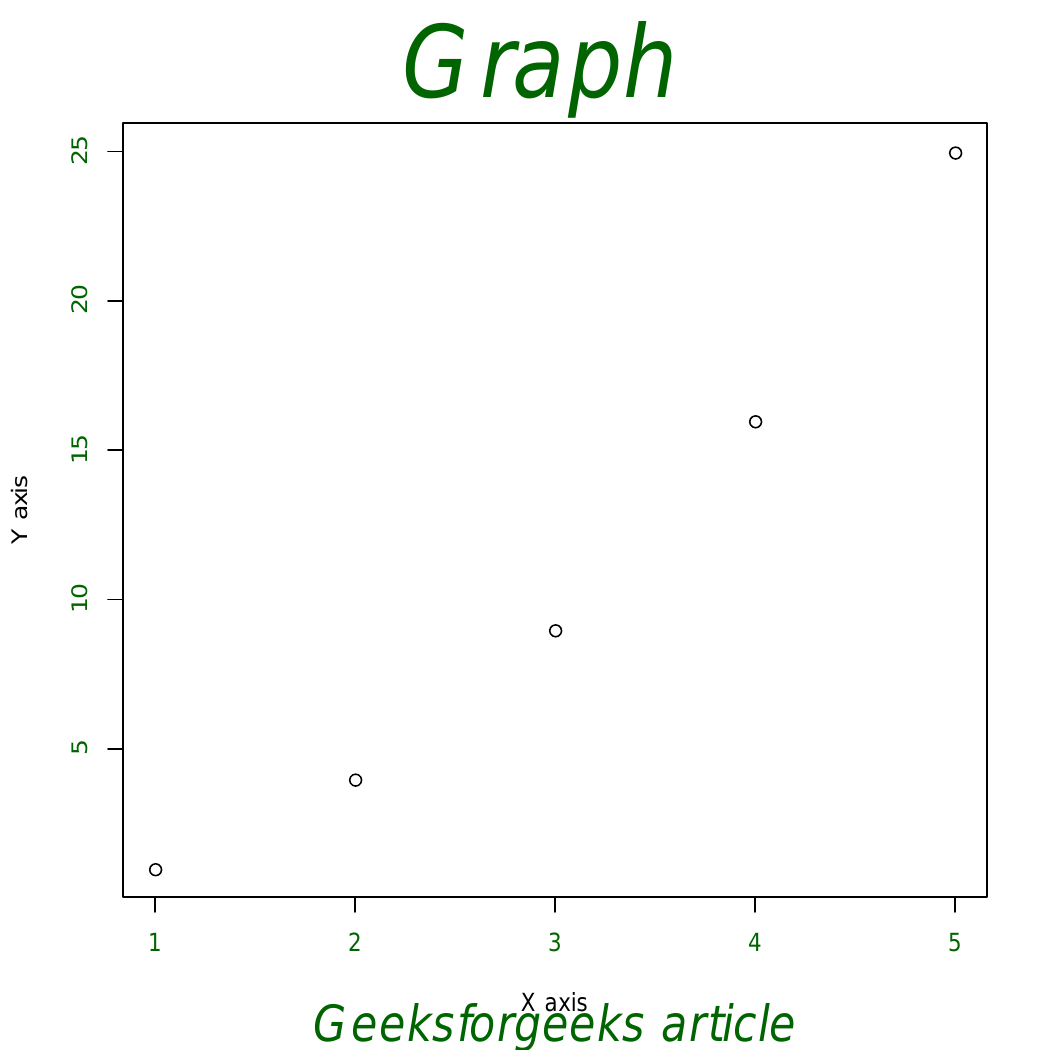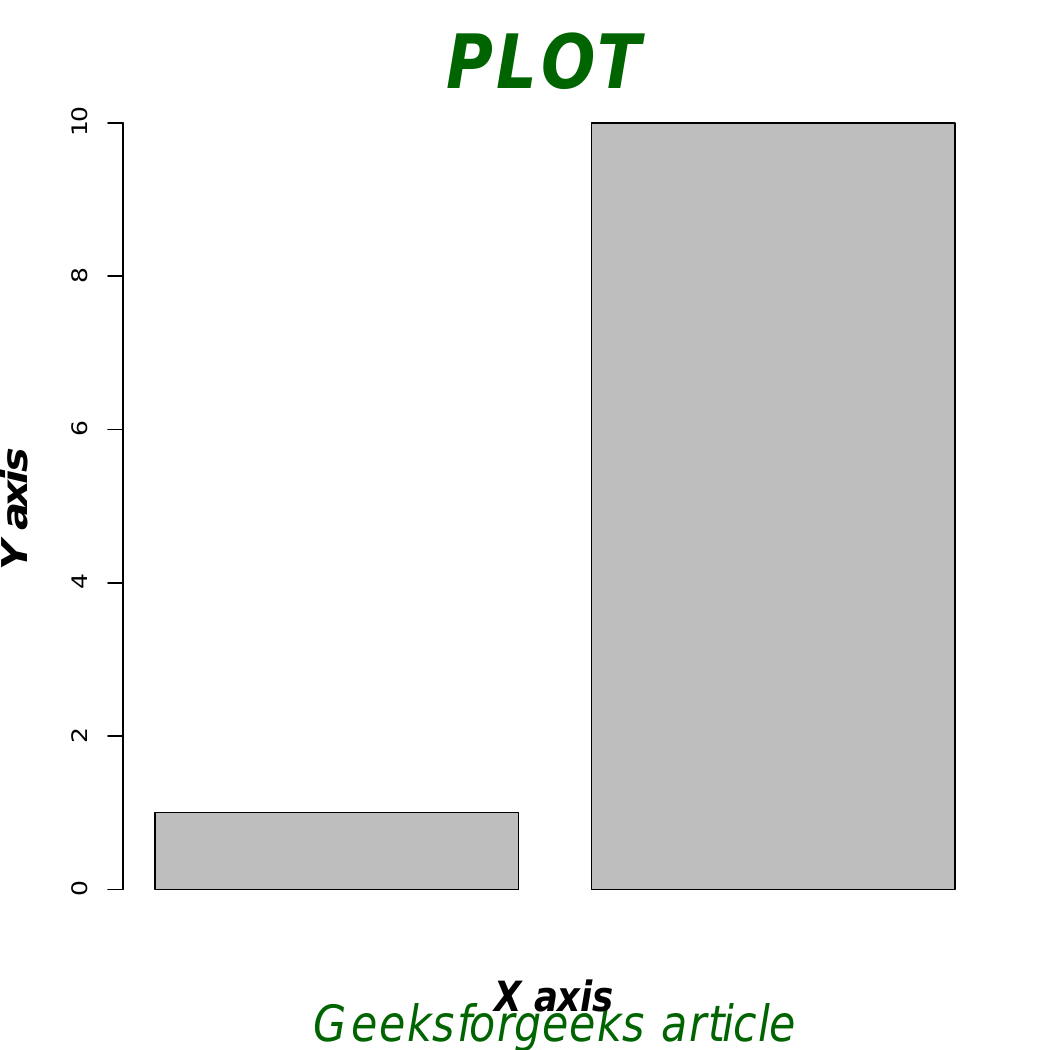# Add Titles to a Graph in R Programming – title() Function

• Last Updated : 20 Jul, 2020

`title()` function in R Language is used to add main title and axis title to a graph. This function can also be used to modify the existing titles.

Syntax:
title(main = NULL, sub = NULL, xlab = NULL, ylab = NULL, …)

Parameters:
main: Main title of the graph
sub: Defines subtitles

Returns: Plot after title addition

Example 1:

 `# R program to add title to a Graph ` ` `  `# Specifying axis values ` `x<-1:5; y<-x*x ` ` `  `# Creating a plot ` `plot``(x, y, main = ``""``, xlab = ``""``, ylab = ``""``, ` `     ``col.axis = ``"darkgreen"``)  ` ` `  `# Calling title() function ` `title``(main = ``"Graph "``, sub = ``"Geeksforgeeks article"``, ` `      ``xlab = ``"X axis"``, ylab = ``"Y axis"``, ` `      ``cex.main = 4,   font.main = 3, col.main = ``"darkgreen"``, ` `      ``cex.sub = 2, font.sub = 3, col.sub = ``"darkgreen"``, ` `      ``col.lab =``"black"` `      ``) `

Output:In above example main title and sub titles are added to the plot. The arguments that can be used to change the font size are as follows:

• cex.main: size for main title
• cex.lab: text size for axis title
• cex.sub: text size of the sub-title

Example 2:

 `barplot``(``c``(1, 10) ) ` `title``(main = ``"PLOT "``, sub = ``"Geeksforgeeks article"``, ` `      ``xlab = ``"X axis"``, ylab = ``"Y axis"``, ` ` `  `  ``# Change the colors ` `  ``col.main=``"darkgreen"``, col.lab=``"black"``, col.sub=``"darkgreen"``, ` ` `  `  ``# Titles in italic and bold ` `  ``font.main = 4, font.lab = 4, font.sub = 3, ` ` `  `  ``# Change font size ` `  ``cex.main = 3, cex.lab = 1.7, cex.sub = 2 ` `  ``) `

Output:My Personal Notes arrow_drop_up
Recommended Articles
Page :